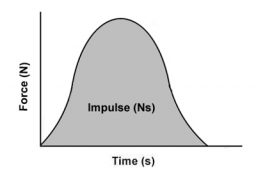# Impulse, force and momentum

A 50 kg mass is sitting on a frictionless surface. An unknown constant force pushes the mass for 2 seconds until the mass reaches a velocity of 3 m/s.

What is the value of the unknown force?
b) through your solutions prove that Impulse and momentum will have an equal value.

F =  75 N

### Step-by-step explanation:Did you find an error or inaccuracy? Feel free to write us. Thank you!

Tips for related online calculators
Need help calculating sum, simplifying, or multiplying fractions? Try our fraction calculator.
Do you want to convert velocity (speed) units?
Do you want to convert time units like minutes to seconds?